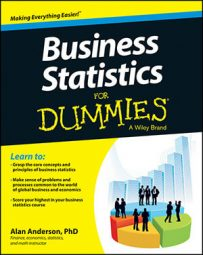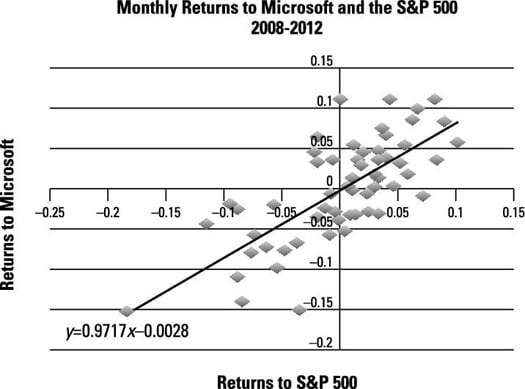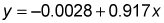A scatter plot (also known as a scatter diagram) shows the relationship between two quantitative (numerical) variables. These variables may be positively related, negatively related, or unrelated:

• Positively related variables indicate that

When one variable increases, the other variable tends to increase.

When one variable decreases, the other variable tends to decrease.

• Negatively related variables indicate that

When one variable increases or decreases, the other variable tends to do the opposite.

• Unrelated variables indicate that

No relationship is seen between the changes in the two variables.

The scatter diagram in the figure shows the relationship between the monthly returns to Microsoft stock and the Standard & Poor’s (S&P) 500 Index from 2008 to 2012:Scatter diagram showing relationship of monthly returns.

Each point on the graph represents the return to Microsoft stock and the return to the S&P 500 Index during a single month. The general direction of these points is from the lower-left corner of the graph to the upper-right corner, indicating that the two variables have a positive relationship.

The graph contains a trend line, which is a straight line designed to come as close as possible to all the points in the diagram. If two variables are positively related, the trend line has a positive slope; similarly, if two variables are negatively related, the trend line has a negative slope. If two variables are unrelated to each other, the trend line has a zero slope (that is, the trend line will be flat).

In the case of Microsoft and the S&P 500 Index, the equation of the trend line isIn this equation, –0.0028 is the intercept (where the trend line crosses the vertical axis) and the slope is 0.917 (how much y changes due to a change in x).

Because the slope of the trend line is positive (0.917), the relationship between the returns to Microsoft stock and the S&P 500 Index is positive. The value of the slope also shows that each 1 percent increase in the returns to the S&P 500 Index increases the return to Microsoft by 0.917 percent, and that each 1 percent decrease in the returns to the S&P 500 Index decreases the return to Microsoft by 0.917 percent.